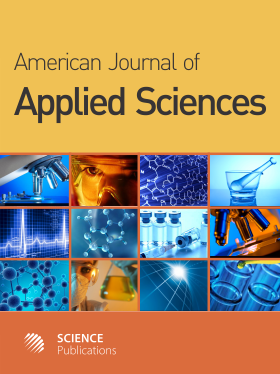Frequency: Continuous
ISSN: 1546-9239 (Print)
ISSN: 1554-3641 (Online)
Research Article Open Access

# β-Spline Estimation in a Semiparametric Regression Model with Nonlinear Time Series Errors

Jinhong You1, Gemai Chen1 and Xian Zhou1
• 1 ,
American Journal of Applied Sciences
Volume 2 No. 9, 2005, 1343-1349

DOI:

Submitted On: 29 January 2005 Published On: 30 September 2005

How to Cite: You, J., Chen, G. & Zhou, X. (2005). β-Spline Estimation in a Semiparametric Regression Model with Nonlinear Time Series Errors. American Journal of Applied Sciences, 2(9), 1343-1349. https://doi.org/10.3844/ajassp.2005.1343.1349

## Abstract

We study the estimation problems for a partly linear regression model with a nonlinear time series error structure. The model consists of a parametric linear component for the regression coefficients and a nonparametric nonlinear component. The random errors are unobservable and modeled by a first-order Markov bilinear process. Based on a B-spline series approximation of the nonlinear component function, we propose a semiparametric ordinary least squares estimator and a semiparametric generalized least squares estimator of the regression coefficients, a least squares estimator of the autoregression parameter for the errors, and a B-spline series estimator of the nonparametric component function. The asymptotic properties of these estimators are investigated and their asymptotic distributions are derived. We also provide a consistent estimator for the asymptotic covariance matrix of the semiparametric generalized least squares estimator of the regression coefficients. Our results can be used to make asymptotically efficient statistical inferences. In addition, a small simulation is conducted to evaluate the performance of the proposed estimators, which shows that the semiparametric generalized least squares estimator of the regression coefficients is more efficient than the semiparametric ordinary least squares estimator.

• 911 Views